output.to from Sideway
Draft for Information Only

# Content

```Second Moment of Mass  Moment of Inertia of a Mass of Body  Radius of Gyraton of Mass of a Body   Moment of Inertia of Mass in Space  Parallel-Axis Thorem of Mass Moment of Inertia  Mass Moment of Inertia of a Three Dimensional Body of Homogenous Material```

# Second Moment of Mass

In general, the second moment of mass in space about an axis is also equal to the summation of the products of the square of the distance between the elemental mass and the reference axis, and the elemental mass over an area. As the second moment of mass is usually refered to the rotation, the distance between the elemental mass and the rotating axis is denoted by r. Imply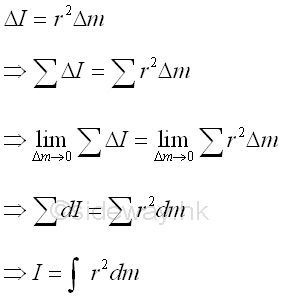## Moment of Inertia of a Mass of BodyConsider a body of mass m, the moment of inertia of mass of the solid body with respect to the axis a can also be determined by dividing the body into elemental mass Δm. The total moment of inertia of mass of the body, which is equal to the sum of all elemental mass, can then be obtained by taking limit as the number of elemental mass approach infinity. Imply## Radius of Gyraton of Mass of a Body

Similarly, the radius of gyraton k of the body is also defined as the distance between  the location of an imaginary total mass of the body and the reference axis such that an equivalent second moment of the mass is created by assuming the imaginary total mass of the body is placed at this distance away from the reference axis. Imply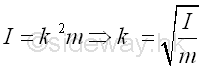Since the inertia of mass is related to the rotation of the body, the imaginary total mass m, used to represent the body, can be located at the perpendicular distance k anywhere away from the axis a for representing the same gyration or the rotation of the body about the axis a.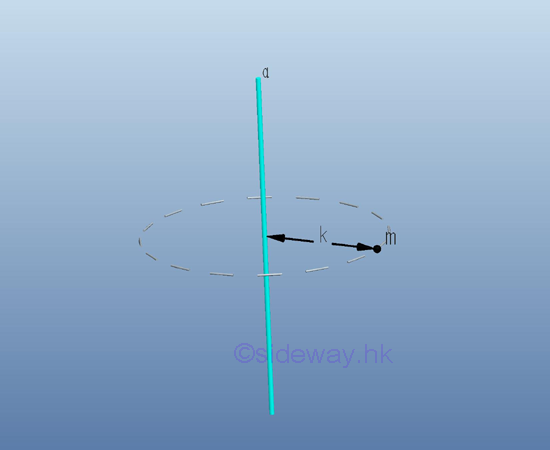## Moment of Inertia of Mass in Space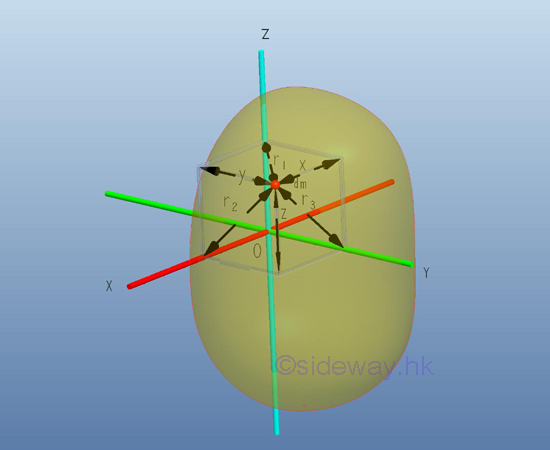Similar to the polar area moment of inertia in a rectangular coordinate system, the mass moment of inertia about an axis in space can also be expressed in terms of the elemental mass about an axis of the rectangular coordinate axes x, y, and z. as in the rectangular area moments of inertia. Imply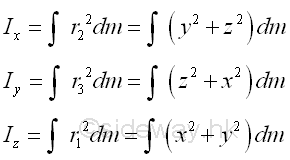## Parallel-Axis Thorem of Mass Moment of Inertia

Consider the same body of mass m with centre of gravity G. Let the rectangular coordinate axes with origin O is XYZ and the parallel centroidal rectangular coordinate axes with origin G, at distance (x,y,z) away from origin O,  is X'Y'Z'. Imply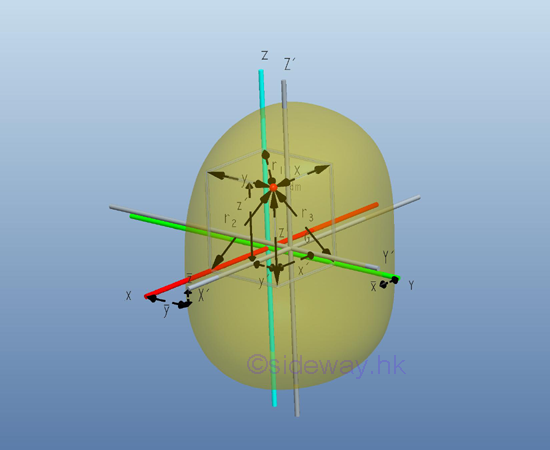Therefore, the distance x,y,z between the elemental mass and the coordinate axes with origin O can be expressed in terms of the distance x', y',z, between the elemental mass and the coordinate axes with origin G. ImplySimilarly the mass moments of inertia of the body about the rectangular coordinate axes with origin O can be expressed in terms of the mass moments of inertia of the body about the parallel centroidal rectangular coordinate axes with origin G also. Imply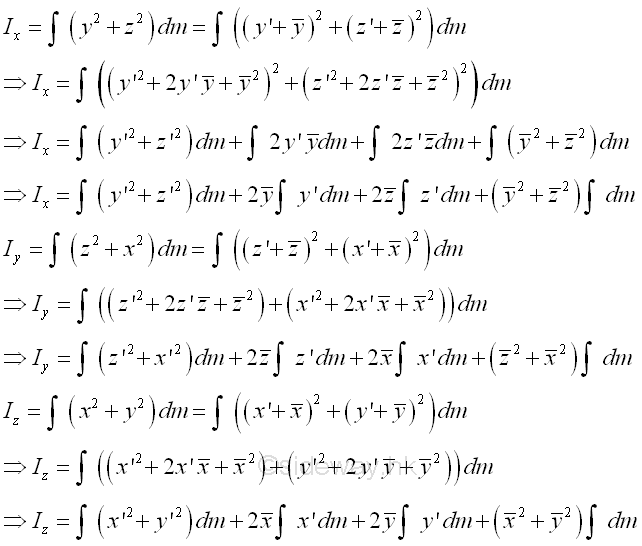The integral of the moment of inertia I of the mass m about the rectangular axis  can be divided into four seperated integrals. The first integral is the centroidal moment of inertia I of the mass. The second and third integrals are the first moment of the mass m about the centroidal axis and the value of the first moment of the mass about the centroidal axis is equal to zero. The fourth integral is equal to the total mass only. Imply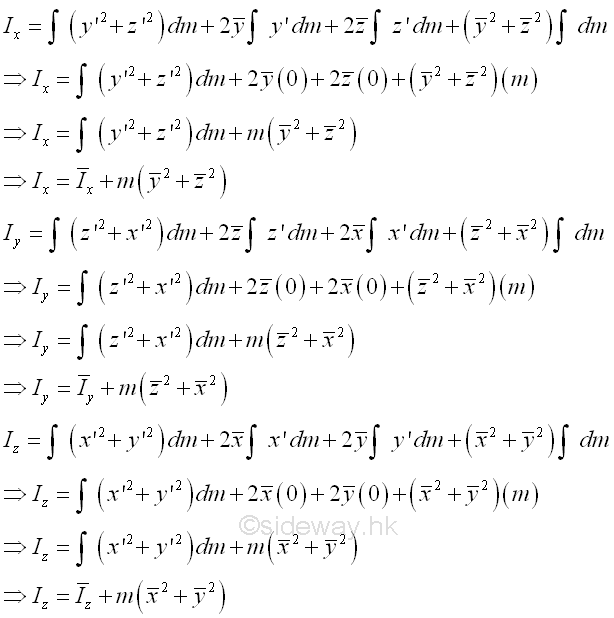The sum of the square of the rectangular coordinate distance from axes is equal to the square of the distance of the perpendicular displacement from the axes. Imply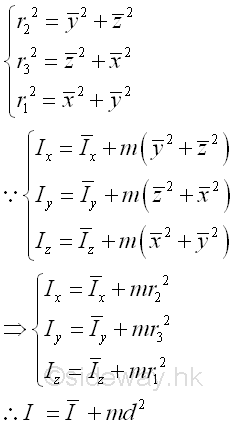Or the mass moment of inertia can be expressed in terms of the radii of gyration. Imply## Mass Moment of Inertia of a Three Dimensional Body of Homogenous Material

For a three-dimensional body made of homomgenous material of density ρ, the elemental mass dm can be expressed in terms of the volume of the three dimensional body. In other words, the mass moment of inertia of a three dimensional body of homogenous material density depends on the shape of the three dimensional body only. Imply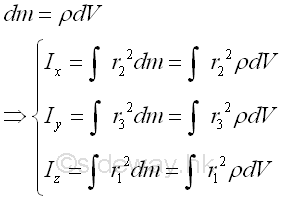ID: 121100085 Last Updated: 14/11/2012 Revision: 0 Ref:References

1. F.P. Beer; E.R. Johnston,Jr.; E.R. Eisenberg, 2004, Vector Mechanics for Engineers: Statics
2. I.C. Jong; B.G. rogers, 1991, Engineering Mechanics: Statics and DynamicsHome 5

Management

HBR 3

Information

Recreation

Culture

Chinese 1097

English 337

Computer

Hardware 151

Software

Application 202

Manim 123

Numeric 19

Programming

Web 285

Unicode 494

HTML 65

CSS 59

ASP.NET 194

OS 391

Python 23

Knowledge

Mathematics

Algebra 29

Geometry 21

Calculus 67

Engineering

Mechanical

Rigid Bodies

Statics 92

Dynamics 37

Control

Physics

Electric 27